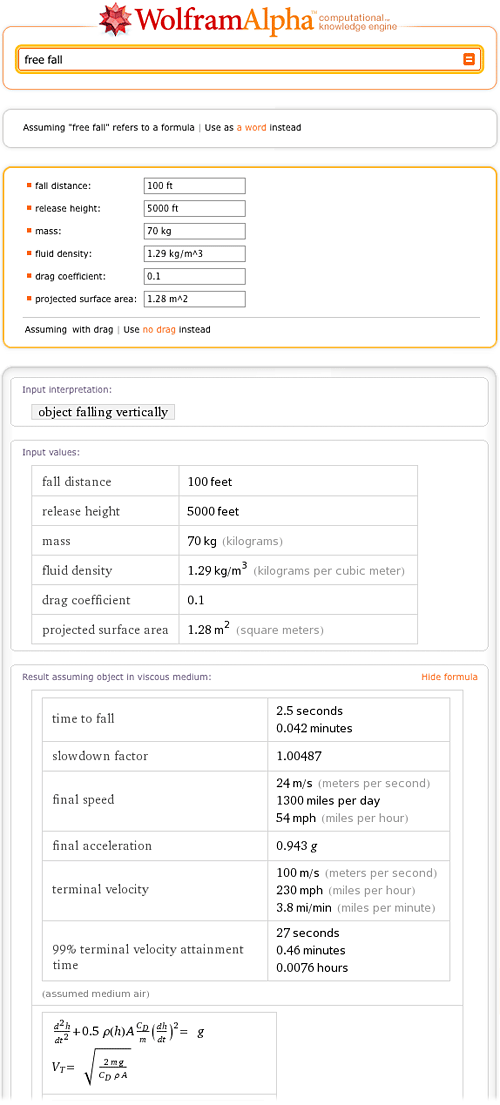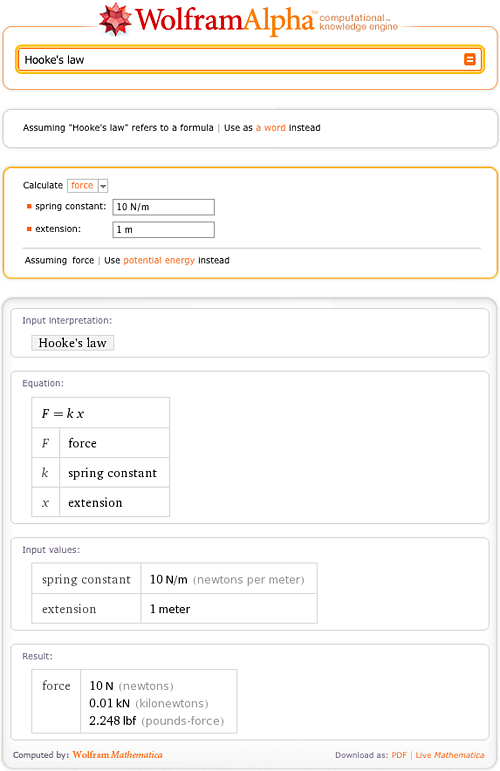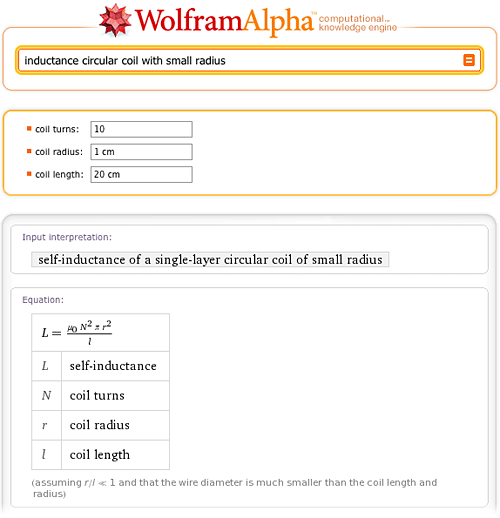TAG: Sums
November 1, 2010–

Wolfram|Alpha is still young and constantly improving. One of the biggest hurdles that our developers are constantly faced with is how to correctly interpret the meaning of general user inputs like “How do I factor an equation?”

Wolfram|Alpha is great at calculating answers that have specific inputs, but when general concepts are given (like “factoring”, for example), it becomes a bit tricky. How would one calculate a concept like that? Let us look at a specific example—Wolfram|Alpha can easily calculate integrals, as long as you ask it to integrate an explicit function. But what happens when you simply ask Wolfram|Alpha to “integrate”? Before, had you given this input, a number of examples using the word “integrate” would have been generated to show how to properly ask Wolfram|Alpha to calculate an integral. But now, when you enter a generic term or question related to a specific math function or formula, it provides a simple query-specific calculator.

For example, given the query “Show me how to integrate”, the following results appear:

Notice that new input fields appear (as well as optional ones in case the integral is to be calculated within a range, and/or with multiple variables). Similarly, notice how the input fields differ depending on the query: More »

June 15, 2010–

Wolfram|Alpha computes things. While the use of computations to predict the outcomes of scientific experiments, natural processes, and mathematical operations is by no means new (it has become a ubiquitous tool over the last few hundred years), the ease of use and accessibility of a large, powerful, and ever-expanding collection of such computations provided by Wolfram|Alpha is.

Virtually all known processes occur in such a way that certain functionals that describe them become extremal. Typically this happens with the action for time dependent processes and quantities such as the free energy for static configurations. The equations describing the extremality condition of a functional are frequently low-order ordinary and/or partial differential equations and their solutions. For example, for a pendulum: Frechet derivative of Integrate[x'[t]^2/2 – Cos[x[t]], {t, -inf, inf}] wrt x[tau]. Unfortunately, if one uses a sufficiently realistic physical model that incorporates all potentially relevant variables (including things like friction, temperature dependence, deformation, and so forth), the resulting equations typically become complicated—so much so that in most cases, no exact closed-form solution can be found, meaning the equations must be solved using numerical techniques. A simple example is provided by free fall from large heights:On the other hand, some systems, such as the force of a simple spring, can be described by formulas involving simple low-order polynomial or rational relations between the relevant problem variables (in this case, Hooke’s law, F = k x):Over the last 200+ years, mathematicians and physicists have found a large, fascinating, and insightful world of phenomena that can be described exactly using these so-called special functions (also commonly known as “the special functions of mathematical physics”), the class of functions that describe phenomena between being difficult and complicated. It includes a few hundred members, and can be viewed as an extension of the so-called elementary functions such as exp(z), log(z), the trigonometric functions, their inverses, and related functions.

Special functions turn up in diverse areas ranging from the spherical pendulum in mechanics to inequivalent representations in quantum field theory, and most of them are solutions of first- or second-order ordinary differential equations. Textbooks often contain simple formulas that correspond to a simplified version of a general physical system—sometimes even without explicitly stating the implicit simplifying assumptions! However, it is often possible to give a more precise and correct result in terms of special functions. For instance, many physics textbooks offer a simple formula for the inductance of a circular coil with a small radius:While Wolfram|Alpha knows (and allows you to compute with) this simple formula, it also knows the correct general result. In fact, if you just ask Wolfram|Alpha for inductance circular coil, you will be simultaneously presented with two calculators: the one you know from your electromagnetics textbook (small-radius approximation) and the fully correct one. And not only can you compute the results both ways (and see that the results do differ slightly for the chosen parameters, but that the difference can get arbitrarily large), you can also click on the second “Show formula” link (near the bottom of the page on the right side) to see the exact result—which, as can be seen, contains two sorts of special functions, denoted E(m) and K(m) and known as elliptic integrals: More »